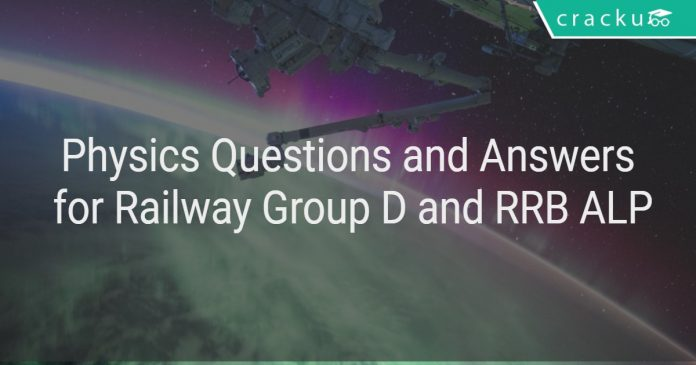# Physics Questions and Answers for Railway Group D and RRB ALP

1
34704Physics Questions and Answers for Railway Group D and RRB ALP

Physics Questions and Answers for Railway Group D and RRB ALP:

General science has very good weightage in railway exam. So, you need to learn physics and biology (science) books of high school. Try to answer this questions from Railways asked in previous year questions papers. Download Railway physics questions and answers PDF.

Download Railways preparation App

Question 1: Which of the following colour deviates the least when passing through a Prism?

a) Violet
b) Green
c) Yellow
d) Red

Question 2:
On which of the following Principles does a Periscope work?

a) Diffraction
b) Dispersion
c) Total Internal Reflection
d) None of these

Question 3: What is the Electronic Configuratin of Sodium in Shell one, two and Three respectively?

a) 2,8
b) 2,8,2
c) 2,8,1
d) 2,8,3

Question 4: Strong Permanent Magnets are used in?

a) Voltmeter
b) Loud Speakers
c) Fridges
d) Both b and c

Question 5: Light Year calculations used by the Astronomers is a measure of which of the following?

a) Temperature
b) Speed
c) Time
d) Distance

Question 6: What happens to the Mass of the Body on the surface of the Moon?

a) Remains Same
b) Decreases
c) Increases
d) Becomes Zero

Question 7: A rocket works on which Law?

a) Angular Momentum
b) Newtons law
c) Linear Momentum
d) Energy

Question 8: The Speed of Sound in Air is independent of which of the following?

a) Temperature of Air
b) Density of Air
c) Moisture in Air
d) Pressure

Question 9: The purpose of the electrostatic Precipitator is to control which of the following Pollutions?

a) Sound Pollution
b) Water Pollution
c) Air pollution
d) Soil Pollution

Question 10: Which of the following devices is widely used to measure Radioactivity?

a) Spectrometer
b) Dynamometer
c) Geiger – Counter
d) Anemometer

Solutions:

1) Answer (d)
2) Answer (c)
3) Answer (c)
4) Answer (d)
5) Answer (d)
6) Answer (a)
7) Answer (a)
8) Answer (d)
9) Answer (c)
10) Answer (c)